# MCAT Physical : Quantum Numbers

## Example Questions

### Example Question #1 : Quantum Numbers

Electronegativity is an important concept in physical chemistry, and often used to help quantify the dipole moment of polar compounds. Polar compounds are different from those compounds that are purely nonpolar or purely ionic. An example can be seen by contrasting sodium chloride, NaCl, with an organic molecule, R-C-OH. The former is purely ionic, and the latter is polar covalent.

When comparing more than one polar covalent molecule, we use the dipole moment value to help us determine relative strength of polarity. Dipole moment, however, is dependent on the electronegativity of the atoms making up the bond. Electronegativity is a property inherent to the atom in question, whereas dipole moment is a property of the bond between them.

For example, oxygen has an electronegativity of 3.44, and hydrogen of 2.20. In other words, oxygen more strongly attracts electrons when in a bond with hydrogen. This leads to the O-H bond having a dipole moment.

When all the dipole moments of polar bonds in a molecule are summed, the molecular dipole moment results, as per the following equation.

Dipole moment = charge * separation distance

A single polar bond, such as the O-H bond described in the passage, is made up of two shared electrons. Before they join together in a bond, what is the first quantum number (n) of the valence electrons of oxygen and hydrogen, respectively?

2 and 2

2 and 1

1 and 1

3 and 1

1 and 2

2 and 1

Explanation:

To determine where the outermost electrons are on a ground state element, we look at the periodic table, and see the row (or period) where that atom resides. This tells us how many electron shells that atom has, which is 2 for oxygen and 1 for hydrogen.

### Example Question #1 : Quantum Numbers

Consider an electron in the 3p subshell. What are its principle (n) and azimuthal (l) quantum numbers?

n = 6, l = 3

n = 3, l = 0

n = 3, l = 1

n = 1, l = 1

n = 3, l = 2

n = 3, l = 1

Explanation:

Finding the principle quantum number, which represents the size of the orbital, is easy. For a 2s or 2p orbital, it would be 2, for a 4s, 4p or 4d orbital it would be 4, etc. In this case, the correct answer is 3 (for 3p). Finding the azimuthal quantum number is slightly more difficult. Values of this number range from 0 to n-1. When n=3, it could theoretically equal 0, 1, or 2. But remember, this number describes the shape of the orbital, and is therefore just another way of stating the letter designation. When l = 0, the orbital is s, when l = 1, the orbital is p, and so on. Here, n = 3 and l = 1, signifying the 3p subshell.

### Example Question #3 : Quantum Numbers

Which of the following sets of quantum numbers could not describe an electron?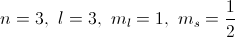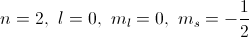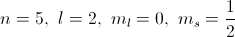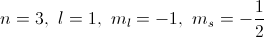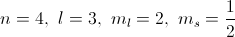Explanation:

The principle quantum number,, must be a positive integer.

The orbital angular quantum number,, ranges fromto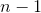, and is also an integer.

The magnetic quantum number,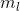, ranges from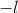to, again is an integer.

The spin quantum number,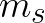, can have only a value of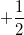or.

Of the choices given, the set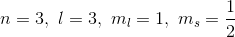because the orbital angular quantum number must be less than the principle quantum number.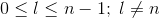### Example Question #1 : Quantum Numbers

Which of the following quantum number sets could describe an atom?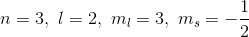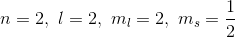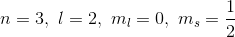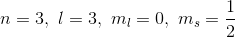Explanation:

This question is testing your knowledge of quantum numbers. The principle quantum number () can be any positive integer value, although it is exceedingly rare that you will see anything greater than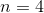.

The azimuthal quantum number () has a range of values from zero to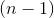, and describes the subshell of the electron in question. In electron configuration, the azimuthal quantum number corresponds to the spdf orbitals.

The magnetic quantum number () has a range of values fromto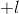, and describes the specific orbital where the electron resides, within the subshell that was described by.

The magnetic spin number (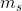) has only two possible values: -and +. It describes the directionality of the electron's spin. In energy diagrams, these are represented by "up" and "down" arrows.

All except one of the given answer choices break rules.Magnetic quantum number must be between positive and negative.Azimuthal quantum number must be less than.Azimuthal quantum number must be less than.

### Example Question #5 : Quantum Numbers

Which of the following is true regarding quantum numbers?

I. The principal quantum number can never be zero

II. It is not possible for two electrons to have the same magnetic quantum number

III. An electron located in a 3d orbital will have an angular momentum number of 2

I and II

I and III

III only

II and III

I and III

Explanation:

Quantum numbers are a type of coordinate system that describe the location and behavior of an electron in an atom. The principal quantum number or,, is the first quantum number. It signifies the energy shell of the electron. The principal quantum number is always greater than 0; therefore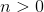. An electron with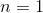signifies that the electron is found within an orbital in the first shell (shell “K”). An electron with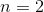is found in the second shell (shell “L”).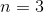is found in the third shell (shell “M”), and so on and so forth. The principal quantum number can never be zero, so statement I is false.

Magnetic quantum number () is the third quantum number. It signifies the orientation of orbitals in space. The s orbital has one possible orientation in space, the p orbital has three possible orientations, and the d orbital has five possible orientations. The rule for magnetic quantum number is as follows: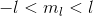is the second quantum number. Therefore, if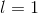(indicating the p orbital), thencan be either -1, 0, or 1 corresponding to the three possible orientations of the p orbital. Recall that each orbital can accommodate only two electrons. This means that both electrons in an orbital will have the samequantum number. Statement II is false.

Angular momentum number,, is the second quantum number and it signifies the type of orbital. A 3d orbital signifies that it is a d orbital that is found in the third shell. The following is true forvalues and the type of orbital.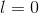indicates an s orbitalindicates a p orbital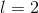indicates a d orbital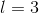indicates an f orbital

Statement III suggests that a d orbital corresponds to the value, which is a true statements.

### Example Question #1 : Quantum Numbers

An electron withequal to __________ can have __________ differentvalues.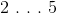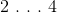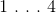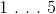Explanation:is the second quantum number, and it signifies the type of orbital housing the electron (for example, spd, or f).is the third quantum number and it signifies the orientation of the orbital in space. The range of values fordepends on the givenvalue. The relationship is as follows:Therefore, if, thencan have three different values: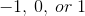.

If, thencan have five different values: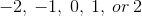.

### Example Question #7 : Quantum Numbers

What can you conclude about an electron withIts orbital can be found in one of three different orientations in space

It is located in either an or a p orbital

Its orbital can be found in shell “K”

It resides in a spherical orbital

Its orbital can be found in one of three different orientations in space

Explanation:

If, then the electron is found in a p orbital. A p orbital can have three different orientations in space; it can found along the x-axis, y-axis, or z-axis (corresponding to the threevalues). An electron withcan be found in any one of these three p orbitals. Note that a shell always has three p orbitals and all of them are oriented in different regions in space. For example, if, then there are three 3p orbitals that are oriented along x, y, and z-axes, respectively.

An s orbital has a spherical shape. Shell “K” is the first shell of an atom (the first energy level). We cannot determine the shell of an electron without knowing the principle quantum number; therefore, we cannot conclude that the electron stated in this question is found in the first shell.

### Example Question #8 : Quantum Numbers

Which of the following is true regarding the quantum numbers of the valence electrons in phosphorous?

All valence electrons in phosphorous have the same set of quantum numbers

Four valence electrons will haveAll valence electrons withcontribute to the paramagnetism of phosphorous

Some of the valence electrons will haveAll valence electrons withcontribute to the paramagnetism of phosphorous
Phosphorous is in the fifth column of the periodic table; therefore, it has five total valence electrons. It is also in the third row of the periodic table, which means that all five valence electrons are located in the third shell (). The valence electrons in phosphorous will be found in either 3s or 3p orbitals. Recall that a shell contains only one s orbital and three p orbitals. Each orbital can contain two electrons; therefore, the first two valence electrons will be found in the 3s orbital and the last three electrons will be found in the 3p orbitals.
Paramagnetic atoms are characterized by the presence of unpaired electrons. As mentioned, phosphorous has five valence electrons that are found in 3s and 3p orbitals. The 3s orbital will have two electrons. This leaves 3 electrons for the three 3p orbitals. According to Hund’s rule, electrons will be first singly distributed amongst orbitals of equal energy before filling in pairs. All three 3p orbitals have the same energy; therefore, the three remaining valence electrons will be singly distributed amongst each of the three 3p orbitals as unpaired electrons. This means that all three valence electrons in the 3p orbitals (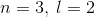) will contribute to the paramagnetism of phosphorous.
As mentioned, all valence electrons will have the same principal quantum number (). Remember that two electrons can never have the same set of quantum numbers. This doesn’t mean that two electrons can’t have the same value for an individual quantum number (for example,for multiple electrons); however, all four quantum numbers can’t be the same for multiple electrons. If, then the electron is found in a p orbital. Only three valence electrons in phosphorous are found in p orbitals; therefore, only these electrons will have.